## Equation Solver 4-in-1 is the ultimate app that helps you solve linear equations in one variable, quadratic equations with real and complex roots, cubic and quartic equations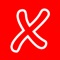# Equation Solver 4in1

by Intemodino Group s.r.o.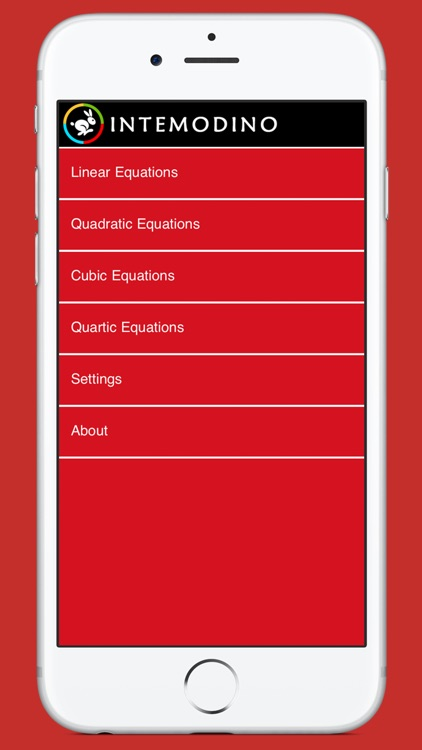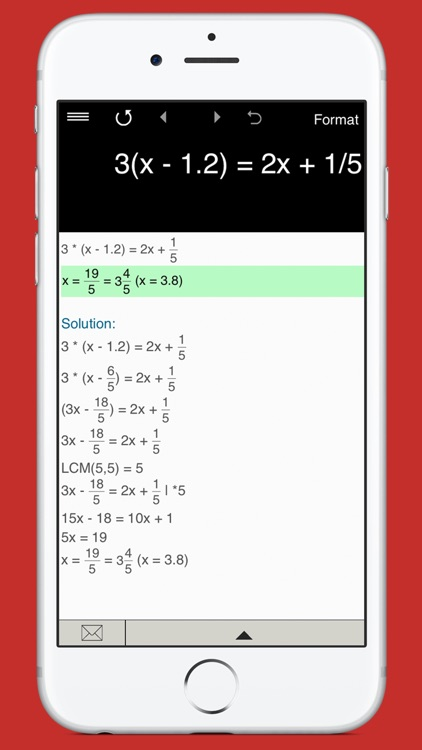Equation Solver 4-in-1 is the ultimate app that helps you solve linear equations in one variable, quadratic equations with real and complex roots, cubic and quartic equations. It's intuitive and easy to use. Just type in any equation you want to solve and the equation calculator will show you the result.### App Details

Version
4.1.5
Rating
(18)
Size
26Mb
Genre
Education Utilities
Last updated
February 10, 2020
Release date
November 9, 2012

### App Screenshots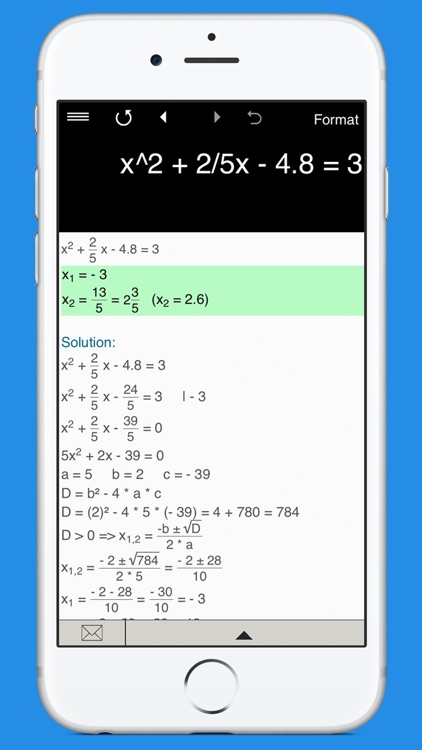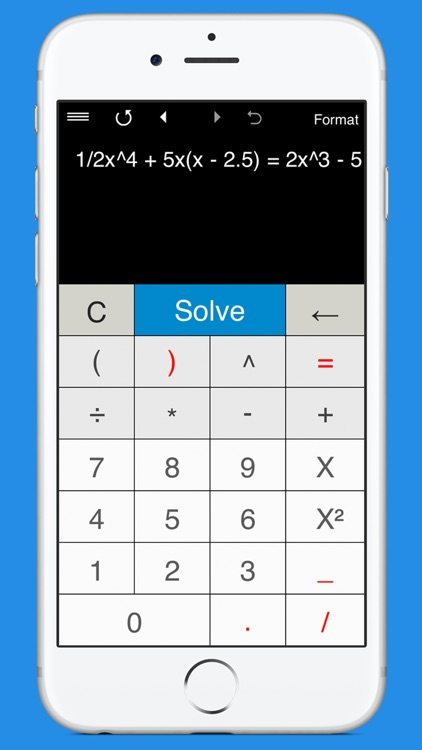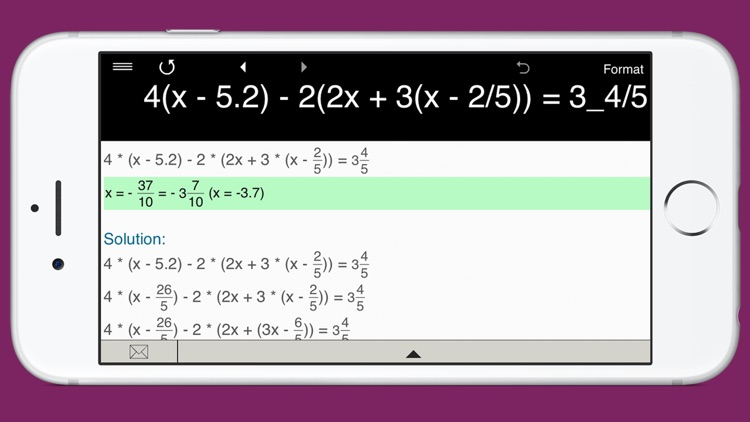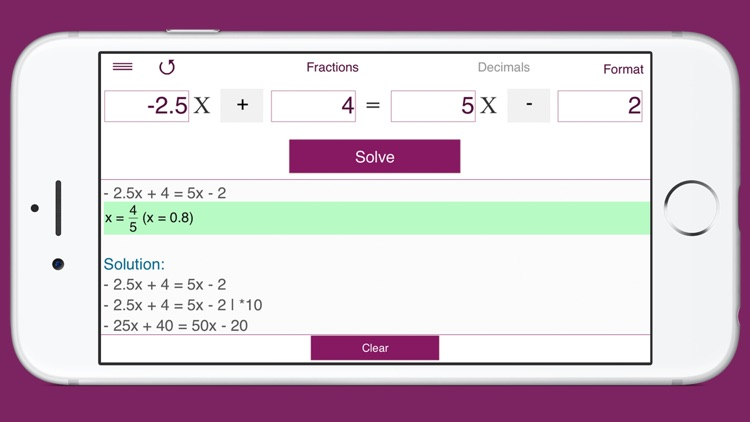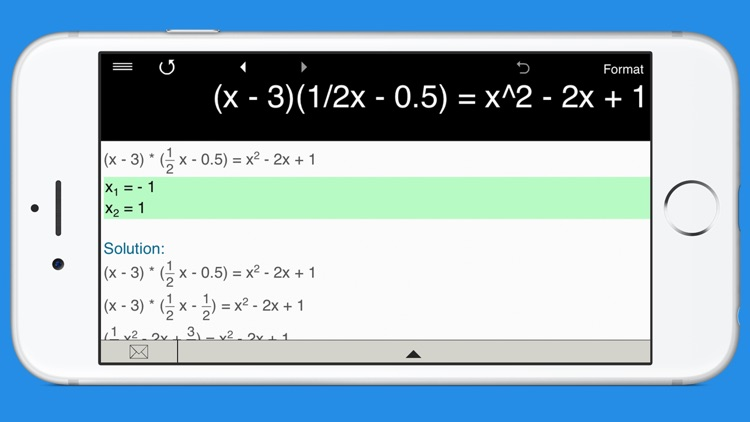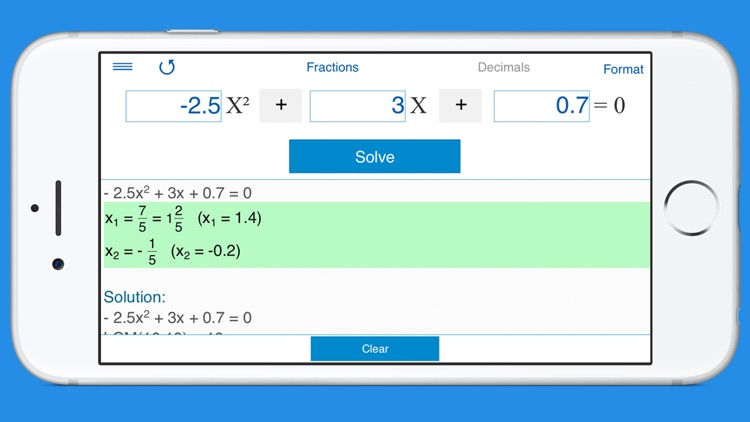### App Store Description

Equation Solver 4-in-1 is the ultimate app that helps you solve linear equations in one variable, quadratic equations with real and complex roots, cubic and quartic equations. It's intuitive and easy to use. Just type in any equation you want to solve and the equation calculator will show you the result.

Everything you need to solve equations or check results!

Features:

• Linear Equation Solver.